# Non parametric Adaptive Importance Sampling (NAIS)¶

The objective is to evaluate a probability from the Non parametric Adaptive Importance Sampling (NAIS) technique.

We consider the four-branch function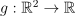defined by:and the input random vector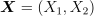which follows the standard 2-dimensional Normal distribution: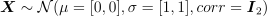We want to evaluate the probability: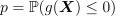First, import the python modules:

import openturns as ot
from openturns.viewer import View
import math


## Create the probabilistic model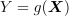¶

Create the input random vector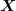:

X = ot.RandomVector(ot.Normal(2))


Create the function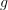from a PythonFunction:

def fourBranch(x):
x1 = x
x2 = x

g1 = 5 + 0.1 * (x1 - x2) ** 2 - (x1 + x2) / math.sqrt(2)
g2 = 5 + 0.1 * (x1 - x2) ** 2 + (x1 + x2) / math.sqrt(2)
g3 = (x1 - x2) + 9 / math.sqrt(2)
g4 = (x2 - x1) + 9 / math.sqrt(2)

return [min((g1, g2, g3, g4))]

g = ot.PythonFunction(2, 1, fourBranch)


Draw the functionto help to understand the shape of the limit state function:

graph = ot.Graph("Four Branch function", "x1", "x2", True, "topright")
drawfunction = g.draw([-8] * 2,  * 2,  * 2)
view = View(graph)In order to be able to get the NAIS samples used in the algorithm, it is necessary to transform the PythonFunction into a MemoizeFunction:

g = ot.MemoizeFunction(g)


Create the output random vector:

Y = ot.CompositeRandomVector(g, X)


## Create the event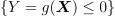¶

threshold = 0.0
myEvent = ot.ThresholdEvent(Y, ot.Less(), threshold)


## Evaluate the probability with the NAIS technique¶

rhoQuantile = 0.1
algo = ot.NAIS(myEvent, rhoQuantile)


Now you can run the algorithm.

algo.run()
result = algo.getResult()
proba = result.getProbabilityEstimate()
print("Proba NAIS = ", proba)
print("Current coefficient of variation = ", result.getCoefficientOfVariation())

Proba NAIS =  6.277611475704379e-06
Current coefficient of variation =  0.11147390478508908


The length of the confidence interval of level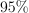is:

length95 = result.getConfidenceLength()
print("Confidence length (0.95) = ", result.getConfidenceLength())

Confidence length (0.95) =  2.7431258600605445e-06


which enables to build the confidence interval:

print(
"Confidence interval (0.95) = [",
proba - length95 / 2,
", ",
proba + length95 / 2,
"]",
)

Confidence interval (0.95) = [ 4.9060485456741064e-06 ,  7.649174405734652e-06 ]


## Draw the NAIS samples used by the algorithm¶

The following manipulations are possible only if you have created a MemoizeFunction that enables to store all the inputs and outputs of the function.

Get all the inputs and outputs wherewere evaluated:

inputNAIS = g.getInputHistory()
outputNAIS = g.getOutputHistory()
nTotal = inputNAIS.getSize()
print("Number of evaluations of g = ", nTotal)

Number of evaluations of g =  4000


Within each step of the algorithm, a sample of size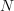is created, where:

N = algo.getMaximumOuterSampling() * algo.getBlockSize()
print("Size of each subset = ", N)

Size of each subset =  1000


You can get the number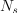of steps with:

Ns = int(nTotal / N)
print("Number of steps = ", Ns)

Number of steps =  4


Now, we can split the initial sample into NAIS samples of size:

listNAISSamples = list()
listOutputNAISSamples = list()
for i in range(Ns):
listNAISSamples.append(inputNAIS[i * N: i * N + N])
listOutputNAISSamples.append(outputNAIS[i * N: i * N + N])


And get all the levels defining the intermediate and final thresholds given by the empirical quantiles of each NAIS output sample:

levels = ot.Point()
for i in range(Ns - 1):


The following graph draws each NAIS sample and the frontier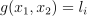where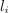is the threshold at the step:

graph = ot.Graph("NAIS samples", "x1", "x2", True, "bottomleft")
graph.setGrid(True)


for i in range(Ns):
cloud = ot.Cloud(listNAISSamples[i])
col = ot.Drawable().BuildDefaultPalette(Ns)
graph.setColors(col)


Add the frontierswhereis the threshold at the step:

gIsoLines = g.draw([-8] * 2,  * 2,  * 2)
dr = gIsoLines.getDrawable(2)
for i in range(levels.getSize()):
dr.setLevels([levels[i]])
dr.setLineStyle("solid")
dr.setLegend(r"$g(X) =$" + str(round(levels[i], 2)))
dr.setLineWidth(3)
dr.setColor(col[i])
view = View(graph)## Draw the frontiers only¶

The following graph enables to understand the progression of the algorithm from the mean value of the initial distribution to the limit state function:

graph = ot.Graph("NAIS thresholds", "x1", "x2", True, "bottomleft")
graph.setGrid(True)
dr = gIsoLines.getDrawable(0)
for i in range(levels.getSize()):
dr.setLevels([levels[i]])
dr.setLineStyle("solid")
dr.setLegend(r"$g(X) =$" + str(round(levels[i], 2)))
dr.setLineWidth(3)